# Reduced Drift-MHD Model

It is convenient to set the normalization scale-length,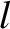, (see Section 4.3) in our reduced drift-MHD model equal to the minor radius of the rational surface,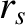. The model evolves four scalar fields. These fields are the (normalized) helical magnetic flux,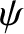, the (normalized) perturbed total plasma pressure,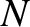, the (normalized) MHD fluid stream-function,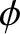, and the (normalized) ion parallel velocity,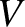. Our four fields have the following definitions: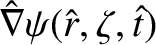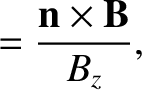(5.1)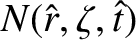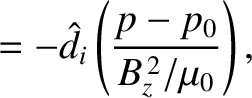(5.2)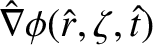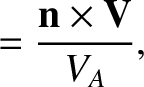(5.3)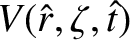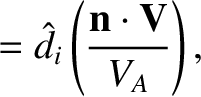(5.4)

where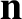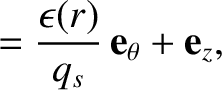(5.5)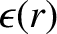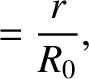(5.6)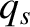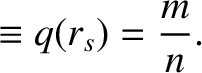(5.7)

[See Equations (4.37), (4.38), (4.41), (4.50), (4.51), (4.53), (4.67), and (4.68).] Here,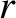,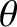,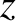are conventional cylindrical coordinates,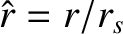,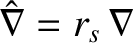,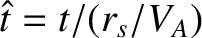,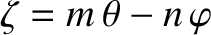a helical angle,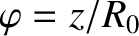a simulated toroidal angle (see Chapter 3),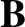the magnetic field-strength,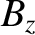the (spatially constant) equilibrium magnetic field-strength,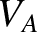the Alfvén speed [see Equation (4.23)],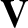the MHD fluid velocity [see Equation (2.321)],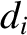the collisionless ion skin-depth [see Equation (4.24)],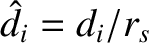,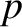the total plasma pressure,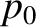the equilibrium total plasma pressure at the rational surface,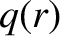the safety-factor profile, andthe simulated major radius of the plasma. The reduced drift-MHD response model also employs the auxiliary fields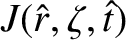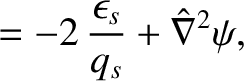(5.8)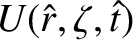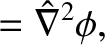(5.9)

where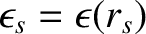.

The reduced drift-MHD model takes the form (see Section 4.5)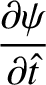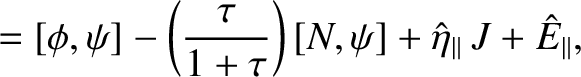(5.10)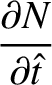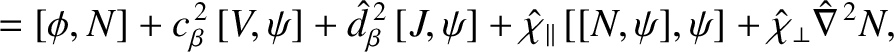(5.11)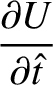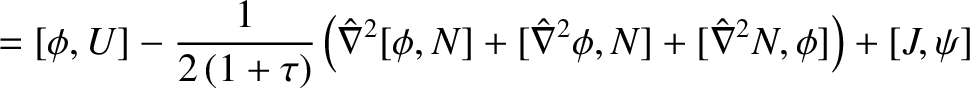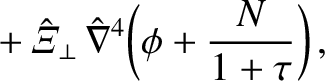(5.12)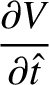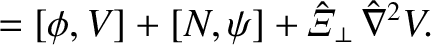(5.13)

Here,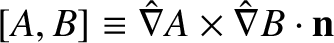,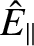is the (normalized) parallel inductive electric field that maintains the parallel plasma current at the rational surface against ohmic decay,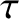the ratio of the electron to the ion equilibrium pressure gradient at the rational surface [see Equation (4.5)],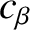a dimensionless measure of the plasma pressure at the rational surface [see Equation (4.65)],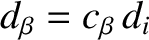the ion sound radius at the rational surface, and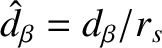. Finally,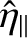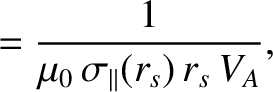(5.14)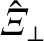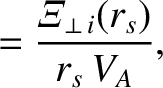(5.15)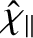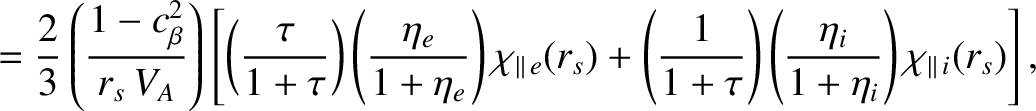(5.16)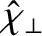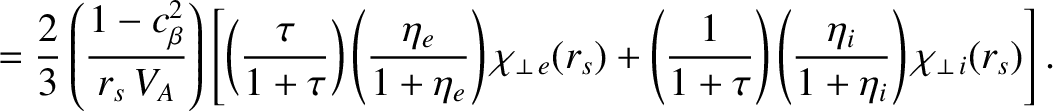(5.17)

are the (normalized) perpendicular (to the equilibrium magnetic field) magnetic flux diffusivity, ion perpendicular momentum diffusivity, effective parallel energy diffusivity, and effective perpendicular energy diffusivity, respectively. [See Equations (4.15), (4.17), (4.18), and (4.19).] Here, the dimensionless parameters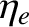and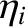are defined in Equations (4.3) and (4.4), respectively. Moreover,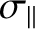,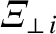,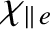,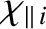,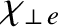, and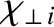are the (classical) parallel electrical conductivity, the ion perpendicular momentum diffusivity, the electron parallel energy diffusivity, the ion parallel energy diffusivity, the electron perpendicular energy diffusivity, and the ion perpendicular energy diffusivity, respectively.